﻿

### Countably enlarging weak barrelledness

#### Abstract

If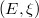$(E, \xi)$ is a locally convex space with dual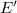$E'$ and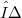$η$ is the coarsest topology finer than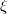$\xi$ such that the dual of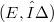$(E, η)$ is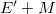$E'+M$ for a given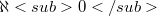$\aleph0$-dimensional subspace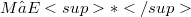$M⊂ E*$ transverse to$E'$, then$η$ is a countable enlargement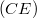$(CE)$ of$\xi$. Here most barrelled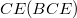$CE(BCE)$ results are optimally extended within the fourteen properties introduced in the 1960s, '70s, '80s, '90s and recently studied in "Reinventing weak barrelledness", et al. If a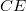$CE$ exists, one exists with none of the fourteen properties. Yet$CE$s that preserve precise subsets of these properties essentially double the stock of distinguishing examples. If a$CE$ exists, must one exist that preserves a given property enjoyed by$\xi$? Under metrizability, the fourteen cases become two: the metrizable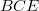$BCE$ question we answered earlier, and the metrizable inductive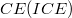$CE(ICE)$ question we answer here (both positively). Without metrizability we are as yet unable to answer Robertson, Tweddle and Yeomans' original$BCE$ question (1979), the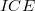$ICE$ question and four others. We give negative answers for the eight remaining general cases, those between$\aleph0$- barrelled and dual locally complete, inclusive, under the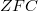$ZFC$-consistent assumption that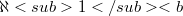$\aleph1 < b$.

DOI Code: 10.1285/i15900932v17p217

Full Text: PDF